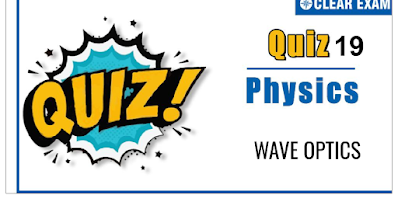## WAVE OPTICS Quiz-19

As per analysis for previous years, it has been observed that students preparing for NEET find Physics out of all the sections to be complex to handle and the majority of them are not able to comprehend the reason behind it. This problem arises especially because these aspirants appearing for the examination are more inclined to have a keen interest in Biology due to their medical background. Furthermore, sections such as Physics are dominantly based on theories, laws, numerical in comparison to a section of Biology which is more of fact-based, life sciences, and includes substantial explanations. By using the table given below, you easily and directly access to the topics and respective links of MCQs. Moreover, to make learning smooth and efficient, all the questions come with their supportive solutions to make utilization of time even more productive. Students will be covered for all their studies as the topics are available from basics to even the most advanced. .

Q1.  The angle of incidence at which reflected light is totally polarized for reflection from air to glass (refractive index 𝑛) is
•   sin-1(𝑛)
•   sin-1 (1/𝑛)
•   tan-1( 1/𝑛 )
•   tan-1(𝑛)
Solution
𝜇𝑚 = tan𝜃𝑝 ⇒ 𝜃𝑝 = tan-1 𝑛

Q2. Unpolarized light of intensity 32𝑊𝑚-2 passes through three polarizers such that transmission axes of the first and second polarizer makes an angle 30° with each other and the transmission axis of the last polarizer is crossed with that of the first. The intensity of final emerging light will be
•   32 𝑊𝑚-2
•   3 𝑊𝑚-2
•   8 𝑊𝑚-2
•   4 𝑊𝑚-2
Solution
Angle between 𝑃1 and 𝑃2= 30° [Given]
Angle between 𝑃2 and 𝑃3 = 𝜃 = 90° − 30° = 60°
The intensity of light transmitted by 𝑃1 is 𝐼1 = 𝐼0 /2 = 32/2 = 16 𝑊 /𝑚2
According to Malus law the intensity of light transmitted by 𝑃2 is 𝐼2 = 𝐼1 cos2 30°
= 16(√3/2 )2 = 12 𝑊/𝑚2
Similarly intensity of light transmitted by 𝑃3 is 𝐼3 = 𝐼2 cos2 𝜃
= 12cos2 60°
= 12( 1/2 )2
= 3 𝑊/𝑚2

Q3.   In the given figure, C is middle point of line𝑆1𝑆2. A monochromatic light of wavelength 𝜆 is incident on slits. The ratio intensity of 𝑆3and 𝑆4 is
•   Zero
•
•   4:1
•   1:4
Solution
As 𝑆3,∆𝑥 = 𝑆1𝑆3 − 𝑆2𝑆3 = 0
∴ ϕ = 2π/λ ∆𝑥 = 0
∴ 𝐼3 = 𝐼0 + 𝐼0 + 2√𝐼0 × 𝐼0(cos0°)
∴ 𝐼3 = 4𝐼0
The path difference at 𝑆4 is
∆𝑥′ = 𝑆3𝑆4 − 𝑆2𝑆4 = 𝑥𝑑/𝐷 (here,𝑥 = 𝜆𝐷 2𝑑 )
= 𝑑 /𝐷 × 𝜆𝐷 /2𝑑 = 𝜆 /2 ∴ ϕ′ = 2π /λ λ/ 2 = π
∴ 𝐼4 = 𝐼0 + 𝐼0 + 2𝐼0 cos𝜋 = 0
∴ 𝐼3 /𝐼4 = 4𝐼0/ 0 = ∞

Q4.  Which of the following cannot be explained on the basis of wave nature of light? IV. Polarization V. Optical activity VI. Photoelectric effect VII. Compton effect
•   (iii) and (iv)
•   (ii) and (iii)
•   (i)and (iii)
•   (ii) and (iv)
Solution
Photoelectric effect and Compton effect cannot be explained on the basis of wave nature of light while polarization and optical activity can be explained

Q5. A slit 5 cm wide is irradiated normally with microwaves of wavelength 1.0 cm. Then the angular spread of the central maximum on either side if incident light is nearly
Solution
Angular spread on either side is θ = λ/𝑎 = 1/5 rad .

Q6.  Intensities of the two waves of light are 𝐼 and 4𝐼. The maximum intensity of the resultant wave after superposition is
•   5 𝐼
•   9 𝐼
• 16 𝐼
•   25 𝐼
Solution
𝐼max= 𝐼1 + 𝐼22 + 2√𝐼1𝐼2 So, 𝐼max = 𝐼 + 4𝐼 + 2√𝐼.4𝐼 = 9𝐼

Q7. The bending of beam of light around corners of obstacles is called

•   Reflection
•   Diffraction
•   Refraction
•   Interference
Solution
Diffraction

Q8. The ratio of maximum and minimum intensities of two sources is 4 :1. The ratio of their amplitudes is
•   1 : 3
• 3 : 1
•   1 : 9
•   1 : 16
Solution
𝐼max / 𝐼min = 4 / 1 (𝑎1 + 𝑎2)2 / (𝑎1 − 𝑎2)2
Or 𝑎1+𝑎2 / 𝑎1−𝑎2 = 2 / 1
Or 𝑎1 + 𝑎2 = 2𝑎1 − 2𝑎2
Or 𝑎1 = 3𝑎2
∴ 𝐼1 / 𝐼2 = 𝑎12 / 𝑎22 = (3𝑎2)2/ 𝑎22 = 9 / 1
∴ 𝑎1 / 𝑎2 = 3 / 1

Q9. . In Young’s double slit experiment, the distance between slits is 0.0344 mm. The wavelength of light used is 600 mm. what is the angular width of a fringe formed on a distant screen?

•
•
•
•
Solution

Q10. A beam of electron is used in an YDSE experiment. The slit width is d . When the velocity of electron is increased, then

•   No interference is observed
•   Fringe width increases
•   Fringe width decreases
•   Fringe width remains same
Solution
As velocity (or momentum) of electron is increased, the wavelength (𝜆 = ℎ/𝑝 ) will decrease. Hence, fringe width will decrease (𝜔 ∝ 𝜆)## Want to know more

Please fill in the details below:

## Latest NEET Articles\$type=three\$c=3\$author=hide\$comment=hide\$rm=hide\$date=hide\$snippet=hide

Name

ltr
item
BEST NEET COACHING CENTER | BEST IIT JEE COACHING INSTITUTE | BEST NEET, IIT JEE COACHING INSTITUTE: WAVE OPTICS QUIZ 19
WAVE OPTICS QUIZ 19